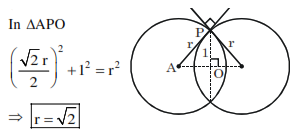# Two circles with equal radii are intersecting at the points (0, 1) and (0, –1).`
Question:

Two circles with equal radii are intersecting at the points (0, 1) and (0, –1). The tangent at the point (0, 1) to one of the circles passes through the centre of the other circle. Then the distance between the centres of these circles is :

1. 1

2. $\sqrt{2}$

3. $2 \sqrt{2}$

4. 2

Correct Option: , 4

Solution:So distance between centres $=\sqrt{2} r=2$